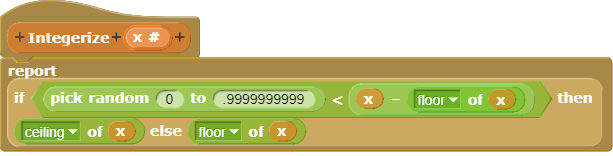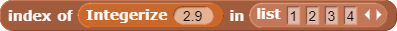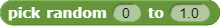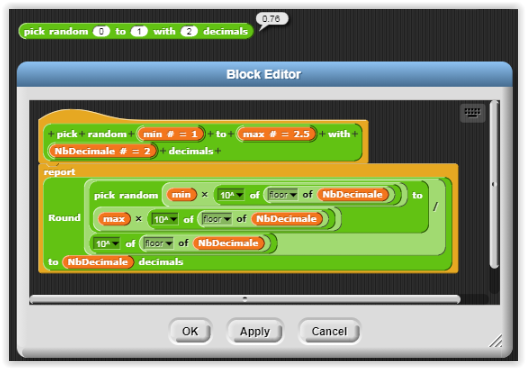# Item -1 of a list

Apropos the discussion in Item 0 of list?

I've been finding it kind of convenient that some languages treat negative indices as counting from the right instead of the left. E.g. item -2 [1, 2, 3, 4] would be 3

I've even enjoyed using systems that evaluate item 2.4 [1, 2, 3, 4] as either 2 or 3 where the odds of it being 2 are .4 or 40%.

I agree! We've been experimenting with such a mechanism. Then I remembered that Jadga and I wrote a paper that's about to be published in the German CSEd journal whose examples rely on negative indices returning nothing. So we took it out again for now until we come up with a good solutionNow I'm curious what was your example that required an empty (I guess that's what 'nothing' means) item being reported?

the "echo" audio-effect.

Good grief. Do use cases for that really come up?

Okay, this is suggesting a crazy idea to me, which I want to call "mixins" because I think it's related to that feature of the (iirc) Lisp Machine Lisp oop system.

Things keep coming up in which people want different behaviors over a bunch of blocks. In this case, non-positive-integer item numbers could mean dictionary behavior, count back from the end, interpolate, etc. Another case that keeps coming up is case-(in)dependent comparisons; we have that for the basic equality test, but really things like CONTAINS and SORT and so on should also have those two modes. A third example is the question of whether FOR and NUMBERS FROM should or shouldn't count downward if end < start. A fourth example is supporting exact rational and complex arithmetic or not.

So, I just thought of this and haven't at all thought about how it might work, but the goal is to have a loadable thing called a mixin, with names like "full numeric tower" or "only count up" or whatever, and what makes it different from just redefining certain blocks is that if two mixins affect the same block, that should work! So, I don't know, item number interpolation that works with exact rational item numbers. Is anything like that even possible?

One special case that comes up often is item (a number between 0 and 1) (a list of two items) where the index represents the probability of choosing the first item instead of the second one.

Though a more general scheme is to use this reporter:for a fractional indexI first saw something like Integerize in modelling populations to deal with non-integer number of people.

I tried to Google this topic and discovered that others have a different interpretation where item 0.75 some_list will report the element that is closest to 75% of the length of the list. But this isn't much of a saving.

P.S. What is the proper way to generate a random number between 0 and 1?Doesn't work

Interesting and maybe doable but as I recall mixins (and the later Common Lisp Object System) they are designed to be a flexible class system and not a way to define different flavours of a function.

Perhaps Aspect-oriented programming is a better fit.

Yeah, I don't think we have a good way to get a truly random floating point value, especially in the case where you want a value in [0,1) rather than in [0,1]. Maybe we need a hidden variant of PICK RANDOM? I'm imagining a block that looks like PICK RANDOM SUCH THAT ( ) < X < ( ) except that the two < signs are actually dropdown menus with choices < and ≤.

Funny. As I was reading the beginning of the article, the part above the table of contents, I thought "Isn't this just metaobject protocols?" and sure enough right under the contents I find Gregor. :~)

Maybe that's the best I could hope for, but the trouble is that you have to anticipate all the kinds of advice that might apply to the base code as you're writing it. That's not much better than just putting all the code there in the first place.

I keep thinking of the Object Logo standard example of multiple inheritance, in which you define a double-length turtle whose FD method does USUAL.FD 2*:DISTANCE, and a dotted turtle that does
PD
USUAL.FD :DISTANCE/2
PU
USUAL.FD :DISTANCE/2
and then you make two derived kinds of turtles, one that inherits from [DOUBLE DOTTED] and another than inherits from [DOTTED DOUBLE], and you draw with them, and they both traverse twice as much distance as the input, but one uses twice as many normal-size dashes and the other uses the same number of double-size dashes. And this isn't a phony demo; you really write the first two special turtle methods without having to think at all of how they'll be combined. It's really magic and it Just Works.

I may be asking for too much, to have that work for every possible retrofitted idea.

PICK RANDOM SUCH THAT makes sense to me.

But

how is [0,1] any easier since 1.0 is treated as an integer? If one could express 1-Number.EPSILON in Snap! that might work.

Yeah, that's how it's easier. We don't have a notation for expressing open intervals -- I mean, intervals without firm endpoints. But the < option would do it.

The other day I needed this for a project:It's not a floating point but

here's a great way to do it

note: I tried doing it with the primitive pick random block, but it didn't work, so I just used the numbers from block.

edit: I found a way to make it not use a list.

That's fine if you don't want a very precise random number. Often getting a random value to within 1/1000 or so is fine, for many practical purposes. And even floating point format in a computer has a limited (although very high) precision, about 15 significant (decimal) digits. But if you imagine that somehow computers could represent all real values (which they can't, because there are uncountably many real numbers, but just imagine), it's an interesting question how you'd find a truly random value.

One of the standard answers to that question is to measure the voltage drop across a (solid-state) diode. This voltage is always very nearly 1.4 volts, but not exactly 1.4 volts. The precise value depends on quantum effects, and changes over time. So if you could build a super-precise voltmeter, and subtract 1.4 from the measured value, you'd get a random real number in a rather small range. (Of course, it's not really a continuously-varying real value, because quantum effects are by definition discrete values, not continuous. But that's true of any physical measurement, and yet we ordinarily think of distances as continuous.)

uh... I don't know what you're trying to say.

Hmm. Okay. Getting a random integer within a given range is straightforward. (Actually, that's a lie; the process for generating one and the mathematics to prove that the ones you generate really are uniformly distributed are quite complicated. But from the user's point of view, it's clear what it means to want/have a random choice from a range of integer values.

Fixed-point fractional random numbers (e.g., to two decimal places), as you understand, is essentially the same problem as random integers, but scaled.

But what about random numbers on a truly continuous range? You can imagine various physical processes, e.g., throw darts at a linear dart board. But how do you do it computationally?

Since 64-bit floating point values are limited to 15 or 16 decimal significant digits, one practical solution is this:

(20 rather than 16 just to be sure to avoid nonrandomness in the low-order bit. But if all the random digits are nines, the result will be rounded to 1.0 by the decimal input algorithm, alas.)

But there are multiword floating point packages, so you can get floating point values to any arbitrary precision, so in principle this is a bad approach even in digital algorithm terms, and no matter what precision you use, it won't give you truly random real values. For that you have to do something non-digital. The diode approach is a textbook example.

Does that help?

ah, thanks. That makes much more sense.

I should add that it's far from obvious (and I'm not even sure it's true) that the values produced by my procedure will be uniformly distributed after rounding. For all I know, some floating point value might be the rounded version of 1000 20-digit numbers, and then next floating value up might be the rounded version of only 999 20-digit numbers.

well, after a bit of coding, and debugging, I found out a way to use the pick random block in it. This way there won't be list length limits, and with huge numbers, it'll be much much quicker.